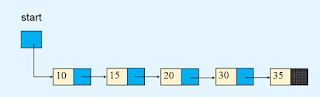A sorted linked list is a list that has been sorted in order while implementing it. let's take an example to understand what is sorted linked list.

let's say we have an empty linked list.first, we insert the value 20 because the linked list is empty so we insert 20 as the first value.after that, we insert value 10, and because the value of the first node is greater than 10 so we insert 10 before the value 20.after that we insert the value 30 and because the 30 is greater than 20 so we insert it at last.after that, we insert values 15 and 15 is greater than 10 but less than 20 so we insert 15 in between the values 10 and 20.after that, we insert value 35, and 35 is greater than 30 so we insert it in last.at last, we insert value 5, and because the value 5 is less than 10 so we insert it before the node that has value 10.so this is the sorted linked list because the linked list is sorted in an order.

### Python program for implementing a sorted linked list.

```class Node(object):

def __init__(self, value):
self.info = value

def __init__(self):
self.start = None
def insert_in_order(self, data):
temp = Node(data)

if self.start is None or data < self.start.info:
self.start = temp
return
p = self.start
while p.link is not None and p.link.info <= data:

def create_list(self):
n = int(input("Enter the number of nodes : "))
if n == 0:
return
for i in range(n):
data = int(input("Enter the element to be inserted : "))
self.insert_in_order(data)

def search(self, x):
if self.start is None:
print("List is empty")
return
p = self.start
position = 1        while p is not None and p.info <= x:
if p.info == x:
break            position += 1            p = p.link

if p is None or p.info != x:
print(x, " not found in list ")
else:
print(x, " is not position", position)

def display_list(self):
if self.start is None:
print("List is empty")
return
print("List is :  ")
p = self.start
while p is not None:
print(p.info, "  ", end='')
print()

################
list.create_list()

while True:
print("1. Display list")
print("2. Insert")
print("3. Search for an element")
print("4. Quit")

option = int(input("Enter your choice : "))

if option == 1:
list.display_list()
elif option == 2:
data = int(input("Enter the element to be inserted : "))
list.insert_in_order(data)
elif option == 3:
data = int(input("Enter the element to be searched : "))
list.search(data)
elif option == 4:
break    else:
print("Wrong option")
print()```